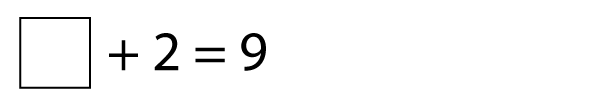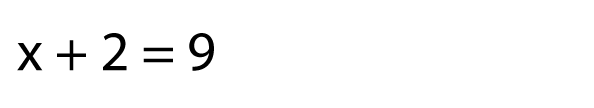What is algebra?

Algebra is like solving a puzzle. Here’s an example of a puzzle we can solve.In algebra, we don't use empty boxes like this. Instead, we use letters. Usually, we use the letters x and y. Letters take up less space than empty boxes!

So this puzzle would become:The letter x represents the empty box, it's the missing number.

Question

x + 2 = 9

x = ?

Solution

? + 2 = 9

7 + 2 = 9

The missing number is 7.